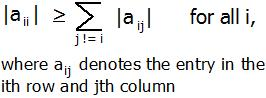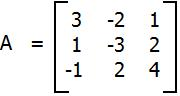# Diagonally Dominant Matrix

• Difficulty Level : Easy
• Last Updated : 19 Aug, 2022

In mathematics, a square matrix is said to be diagonally dominant if for every row of the matrix, the magnitude of the diagonal entry in a row is larger than or equal to the sum of the magnitudes of all the other (non-diagonal) entries in that row. More precisely, the matrix A is diagonally dominant ifFor example, The matrixis diagonally dominant because
|a11| ≥ |a12| + |a13| since |+3| ≥ |-2| + |+1|
|a22| ≥ |a21| + |a23| since |-3| ≥ |+1| + |+2|
|a33| ≥ |a31| + |a32| since |+4| ≥ |-1| + |+2|
Given a matrix A of n rows and n columns. The task is to check whether matrix A is diagonally dominant or not.

Examples :

```Input : A = { { 3, -2, 1 },
{ 1, -3, 2 },
{ -1, 2, 4 } };
Output : YES
Given matrix is diagonally dominant
because absolute value of every diagonal
element is more than sum of absolute values
of corresponding row.

Input : A = { { -2, 2, 1 },
{ 1, 3, 2 },
{ 1, -2, 0 } };
Output : NO```

The idea is to run a loop from i = 0 to n-1 for the number of rows and for each row, run a loop j = 0 to n-1 find the sum of non-diagonal element i.e i != j. And check if diagonal element is greater than or equal to sum. If for any row, it is false, then return false or print “No”. Else print “YES”.

Implementation:

## C++

 `// CPP Program to check whether given matrix` `// is Diagonally Dominant Matrix.` `#include ` `#define N 3` `using` `namespace` `std;`   `// check the given  matrix is Diagonally` `// Dominant Matrix or not.` `bool` `isDDM(``int` `m[N][N], ``int` `n)` `{` `    ``// for each row` `    ``for` `(``int` `i = 0; i < n; i++)` `   ``{        `   `        ``// for each column, finding sum of each row.` `        ``int` `sum = 0;` `        ``for` `(``int` `j = 0; j < n; j++)             ` `            ``sum += ``abs``(m[i][j]);        `   `        ``// removing the diagonal element.` `        ``sum -= ``abs``(m[i][i]);`   `        ``// checking if diagonal element is less ` `        ``// than sum of non-diagonal element.` `        ``if` `(``abs``(m[i][i]) < sum) ` `            ``return` `false``; ` `       `  `    ``}`   `    ``return` `true``;` `}`   `// Driven Program` `int` `main()` `{` `    ``int` `n = 3;` `    ``int` `m[N][N] = { { 3, -2, 1 },` `                    ``{ 1, -3, 2 },` `                    ``{ -1, 2, 4 } };`   `    ``(isDDM(m, n)) ? (cout << ``"YES"``) : (cout << ``"NO"``);`   `    ``return` `0;` `}`

## Java

 `// JAVA Program to check whether given matrix` `// is Diagonally Dominant Matrix.` `import` `java.util.*;`   `class` `GFG {` `    `  `    ``// check the given  matrix is Diagonally` `    ``// Dominant Matrix or not.` `    ``static` `boolean` `isDDM(``int` `m[][], ``int` `n)` `    ``{` `        ``// for each row` `        ``for` `(``int` `i = ``0``; i < n; i++)` `        ``{        ` `     `  `            ``// for each column, finding ` `            ``//sum of each row.` `            ``int` `sum = ``0``;` `            ``for` `(``int` `j = ``0``; j < n; j++)             ` `                ``sum += Math.abs(m[i][j]);        ` `     `  `            ``// removing the diagonal element.` `            ``sum -= Math.abs(m[i][i]);` `     `  `            ``// checking if diagonal element is less ` `            ``// than sum of non-diagonal element.` `            ``if` `(Math.abs(m[i][i]) < sum) ` `                ``return` `false``; ` `       `  `        ``}`   `        ``return` `true``;` `    ``}`   `    ``/* Driver program to test above function */` `    ``public` `static` `void` `main(String[] args) ` `    ``{` `        ``int` `n = ``3``;` `        ``int` `m[][] = { { ``3``, -``2``, ``1` `},` `                      ``{ ``1``, -``3``, ``2` `},` `                      ``{ -``1``, ``2``, ``4` `} };` `     `  `        ``if` `(isDDM(m, n))` `             ``System.out.println(``"YES"``) ;` `        ``else`  `            ``System.out.println(``"NO"``);` `    `  `    ``}` `}`   `// This code is contributed by  Arnav Kr. Mandal.`

## Python3

 `# Python Program to check` `# whether given matrix is ` `# Diagonally Dominant Matrix.`   `# check the given  ` `# matrix is Diagonally ` `# Dominant Matrix or not.` `def` `isDDM(m, n) :`   `    ``# for each row` `    ``for` `i ``in` `range``(``0``, n) :         ` `    `  `        ``# for each column, finding` `        ``# sum of each row.` `        ``sum` `=` `0` `        ``for` `j ``in` `range``(``0``, n) :` `            ``sum` `=` `sum` `+` `abs``(m[i][j])     `   `        ``# removing the ` `        ``# diagonal element.` `        ``sum` `=` `sum` `-` `abs``(m[i][i])`   `        ``# checking if diagonal ` `        ``# element is less than ` `        ``# sum of non-diagonal` `        ``# element.` `        ``if` `(``abs``(m[i][i]) < ``sum``) :` `            ``return` `False`   `    ``return` `True`   `# Driver Code` `n ``=` `3` `m ``=` `[[ ``3``, ``-``2``, ``1` `],` `    ``[ ``1``, ``-``3``, ``2` `],` `    ``[ ``-``1``, ``2``, ``4` `]]`   `if``((isDDM(m, n))) :` `    ``print` `(``"YES"``)` `else` `:` `    ``print` `(``"NO"``)`   `# This code is contributed by ` `# Manish Shaw(manishshaw1)`

## C#

 `// C# Program to check whether given matrix` `// is Diagonally Dominant Matrix.` `using` `System;`   `class` `GFG {` `    `  `    ``// check the given matrix is Diagonally` `    ``// Dominant Matrix or not.` `    ``static` `bool` `isDDM(``int` `[,]m, ``int` `n)` `    ``{` `        ``// for each row` `        ``for` `(``int` `i = 0; i < n; i++)` `        ``{ ` `    `  `            ``// for each column, finding ` `            ``//sum of each row.` `            ``int` `sum = 0;` `            ``for` `(``int` `j = 0; j < n; j++)         ` `                ``sum += Math.Abs(m[i, j]);     ` `    `  `            ``// removing the diagonal element.` `            ``sum -= Math.Abs(m[i, i]);` `    `  `            ``// checking if diagonal element is less ` `            ``// than sum of non-diagonal element.` `            ``if` `(Math.Abs(m[i,i]) < sum) ` `                ``return` `false``; ` `        `  `        ``}`   `        ``return` `true``;` `    ``}`   `    ``// Driver program ` `    ``public` `static` `void` `Main() ` `    ``{` `        ``int` `n = 3;` `        ``int` `[,]m = { { 3, -2, 1 },` `                    ``{ 1, -3, 2 },` `                    ``{ -1, 2, 4 } };` `    `  `        ``if` `(isDDM(m, n))` `            ``Console.WriteLine(``"YES"``) ;` `        ``else` `            ``Console.WriteLine(``"NO"``);` `    `  `    ``}` `}`   `// This code is contributed by Vt_m.`

## PHP

 ``

## Javascript

 ``

Output

`YES`

Time Complexity: O(N2)
Auxiliary Space: O(1), since no extra space has been taken.

My Personal Notes arrow_drop_up
Recommended Articles
Page :